# GSEB Solutions Class 6 Maths Chapter 14 Practical Geometry Ex 14.5

Gujarat Board GSEB Textbook Solutions Class 6 Maths Chapter 14 Practical Geometry Ex 14.5 Textbook Questions and Answers.

## Gujarat Board Textbook Solutions Class 6 Maths Chapter 14 Practical Geometry Ex 14.5

Question 1.
Draw $$\overline{\mathrm{AB}}$$ of length 7.3 cm and find its axis of symmetry.
Note: The perpendicular bisector of a line segment is its axis of symmetry.
Solution:
Steps of construction:
Step I: Draw line segment $$\overline{\mathrm{AB}}$$ = 7.3 cm.
Step II: With centres A and B and radius more than half of AB, draw two arcs that intersect each other at P and Q.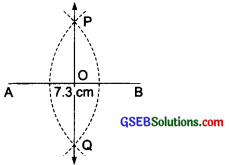Step III: Join P and Q.
Thus, PQ is the axis of symmetry of $$\overline{\mathrm{AB}}$$.

Question 2.
Draw a line segment of length 9.5 cm and construct its perpendicular bisector.
Solution:
Steps of construction:
Step I: Draw a line segment AB = 9.5 cm.
Step II: With A and B as centres and radius more than half of $$\overline{\mathrm{AB}}$$, draw two arcs on either side of AB which intersects each other at P and Q.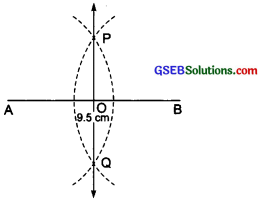Step III: Join P and Q.
Thus, PQ is the required perpendicular bisector of $$\overline{\mathrm{AB}}$$.Question 3.
Draw the perpendicular bisector of $$\overline{\mathrm{XY}}$$ whose length is 10.3 cm.
(a) Take any point P on the bisector drawn. Examine whether PX = PY.
(b) If M is the mid-point of $$\overline{\mathrm{XY}}$$, what can you say about the lengths MX and XY?
Solution:
Steps of construction:
Step I: Draw a line segment $$\overline{\mathrm{XY}}$$ = 10.3 cm
Step II: With X and Y as centres and radius more than half of XY, draw two arcs on either side of XY which intersect each other at A and B.
Step III: join A and B.
Thus, AB is ⊥ $$\overline{\mathrm{XY}}$$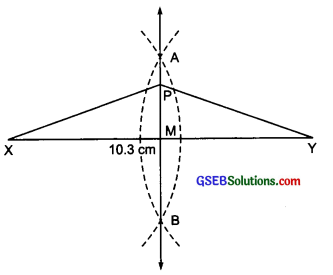Step IV: Mark a point ‘P’ on AB and join PX and PY.
(a) On measuring $$\overline{\mathrm{PX}}$$ and $$\overline{\mathrm{PY}}$$ (using a divider) we get that $$\overline{\mathrm{PX}}$$ = $$\overline{\mathrm{PY}}$$ .
(b) The mid-point of XY is M. On measuring,
we have that $$\overline{\mathrm{XM}}$$ = $$\overline{\mathrm{MY}}$$ = $$\frac { 1 }{ 2 }$$ XY.

Question 4.
Draw a line segment of length 12.8 cm. Using compasses, divide it into four equal parts. Verify by actual measurement.
Solution:
Steps of construction:
Step I: Draw a line segment $$\overline{\mathrm{AB}}$$ = 12.8 cm.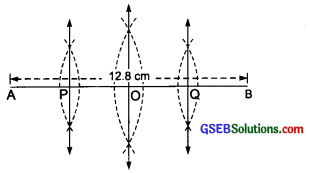Step II: Draw perpendicular bisector of AB, which meets $$\overline{\mathrm{AB}}$$ at O. (i.e. O is the mid-point of $$\overline{\mathrm{AB}}$$)
Step III: Draw perpendicular bisector of $$\overline{\mathrm{AO}}$$, which meet $$\overline{\mathrm{AB}}$$ at P. {i.e. P is mid-point of $$\overline{\mathrm{AO}}$$)
Step IV: Now, draw perpendicular bisector of $$\overline{\mathrm{BO}}$$, which meets AB at Q. (i.e. Q is the mid-point of OB)
Step V: The line segment is divided into 4 equal parts by the points P, 0 and Q.
Step VI: By actual measurement, we have:
$$\overline{\mathrm{AP}}$$ = $$\overline{\mathrm{PO}}$$ = $$\overline{\mathrm{OQ}}$$ = $$\overline{\mathrm{QB}}$$ = 3.2 cmQuestion 5.
With $$\overline{\mathrm{PQ}}$$ of length 6.1 cm as diameter, draw a circle.
Solution:
Steps of construction:
Step I: Draw a line segment $$\overline{\mathrm{XY}}$$ =6.1 cm.
Step II: Draw the perpendicular bisector of PQ which meets $$\overline{\mathrm{XY}}$$ at O. (i.e. O is the mid-point of PQ).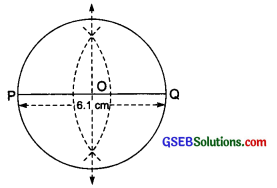Step III: With centre O and $$\overline{\mathrm{OP}}$$ or $$\overline{\mathrm{OQ}}$$ as radius, draw a circle passing through P and Q.
The circle having $$\overline{\mathrm{XY}}$$ as the diameter is the required circle.

Question 6.
Draw a circle with centre C and radius 3.4 cm. Draw any chord $$\overline{\mathrm{AB}}$$.Construct the perpendicular bisector of $$\overline{\mathrm{AB}}$$ and examine if it passes through C.
Solution:
Steps of construction:
Step I: Mark a point C on a paper.
Step II: With centre ‘C’ and radius 3.4 cm, draw a circle.
Step III: Draw a chord $$\overline{\mathrm{AB}}$$.
Step IV: Draw perpendicular bisector of $$\overline{\mathrm{AB}}$$.
We find that l (the perpendicular bisector of $$\overline{\mathrm{AB}}$$) passes through the centre of the circle.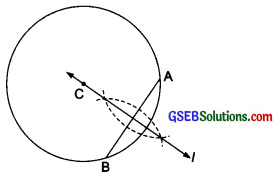Question 7.
Repeat question 6, if $$\overline{\mathrm{AB}}$$ happens to be a diameter.
Solution:
Steps of construction:
Step I: Mark a point ‘C’ on a paper.
Step II: With centre C and radius 3.4 cm, draw a circle.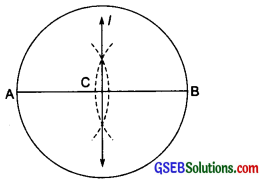Step III: Draw a diameter $$\overline{\mathrm{AB}}$$ of the circle.
Step IV: Draw the perpendicular bisector ‘l’ of $$\overline{\mathrm{AB}}$$. We find that l passes through C and C is the mid-point of AB.Question 8.
Draw a circle of radius 4 cm. Draw any two of its chords. Construct the perpendicular bisectors of these chords. Where do they meet?
Solution:
Steps of construction:
Step I: Mark a point O on a paper.
Step II: With centre O and radius 4 cm, draw a circle.
Step III: Draw two chords $$\overline{\mathrm{AB}}$$ and $$\overline{\mathrm{CD}}$$.
Step IV: Draw perpendicular bisector ‘l’ of $$\overline{\mathrm{AB}}$$.
Step V: Draw perpendicular bisector ‘M’ of $$\overline{\mathrm{CD}}$$.
Step VI: Produce l and m to meet each other. We find that l and m meet at O, the centre of the circle.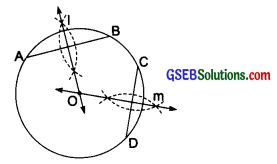Question 9.
Draw any angle with vertex O. Take a point A on one of its arms and B on another such that OA = OB. Draw the perpendicular bisectors of $$\overline{\mathrm{OA}}$$ and $$\overline{\mathrm{OB}}$$. Let them meet at P. Is PA = PB?
Solution:
Steps of construction:
Step I: Mark a point O on a paper.
Step II: Draw an angle ∠XOY, having a vertex at O.
Step III: Mark a point A on $$\overrightarrow{\mathrm{OX}}$$ and another point B on $$\overrightarrow{\mathrm{OY}}$$, such that $$\overline{\mathrm{OA}}$$ = $$\overline{\mathrm{OB}}$$.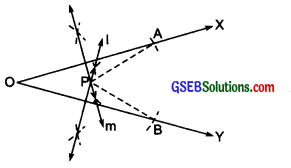Step IV: Draw l, the perpendicular bisector of $$\overline{\mathrm{OB}}$$ .
Step V: Draw m, the perpendicular bisector of $$\overline{\mathrm{OA}}$$
Step VI: Mark the intersecting points of l and m as P.
Step VII: Join $$\overline{\mathrm{PA}}$$ and $$\overline{\mathrm{PB}}$$, measure them with the help of a divider.
Step VIII: On measuring, we find $$\overline{\mathrm{PA}}$$ = $$\overline{\mathrm{PB}}$$